# MA.912.AR.1.7Export Print
Rewrite a polynomial expression as a product of polynomials over the real number system.

### Examples

Example: The expression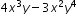is equivalent to the factored form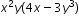.

Example: The expression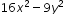is equivalent to the factored form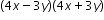.

### Clarifications

Clarification 1: Within the Algebra 1 course, polynomial expressions are limited to 4 or fewer terms with integer coefficients.
General Information
Subject Area: Mathematics (B.E.S.T.)
Strand: Algebraic Reasoning
Status: State Board Approved

## Benchmark Instructional Guide

• Expression
• Polynomial

### Vertical Alignment

Previous Benchmarks

Next Benchmarks

### Purpose and Instructional Strategies

In grade 8, students rewrote binomial algebraic expressions as a common factor times a binomial. In Algebra I, students rewrite polynomials, up to 4 terms, as a product of polynomials over the real numbers. In later grades, students will rewrite polynomials as a product of polynomials over the real and complex number systems.
• Instruction includes special cases such as difference of squares and perfect square trinomials.
• Instruction builds upon student prior knowledge of factors, including greatest common factors.
• Instruction includes the student understanding that factoring is the inverse of multiplying polynomial expressions.
• Instruction includes the use of models, manipulatives and recognizing patterns when factoring. o
• Sum-Product Pattern
• The expression $x$2 + 7$x$ + 10 can be written as ($x$ + 5)($x$ + 2) since 5 + 2 = 7 and 5(2) =  10.
• Factor by Grouping
• The expression $x$3+ 7$x$2+ 2$x$ + 14 can be grouped into two binomials and rewritten as  ($x$3+ 7$x$2) + (2$x$ + 14). Each binomials can be factored and rewritten as $x$2 ($x$ + 7) + 2($x$ + 7) resulting in  the same factor and the factored form as ($x$2 + 2)($x$ + 7)
• A-C Method
• When factoring trinomials $a$$x$2 + $b$$x$+ $c$ , multiply $a$ and $c$, then determine factor pairs of   the  product. Using the factor pair that add to $b$ and multiply to $c$, rewrite the middle term and  then factor by grouping.
• For example, given 2$x$2 + $x$ − 6  and that $a$$c$ = −12, one can determine that two numbers that add to 1 and multiple to -12 are 4 and -3. This information can be used to rewrite the given quadratic as 2$x$2 + 4$x$ − 3$x$ − 6 . Then, using factor by grouping the expression is equivalent to (2$x$2 + 4$x$) - (3$x$ + 6)  which is equivalent to 2$x$($x$ + 2) − 3($x$ + 2) which is equivalent to the factored form (2$x$ − 3)($x$ + 2).
• Box Method
• To factor $a$$x$2 + $b$$x$ + $c$  the general box method is shown below.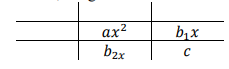• For example, to factor 2$x$2 - 9$x$ - 5  the box method is shown below.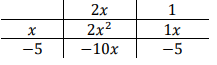• Area Model (Algebra tiles)
• The factorization of  2$x$2 - 9$x$ - 5 using algebra tiles is shown below.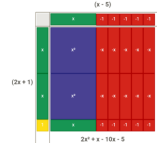### Common Misconceptions or Errors

• Students may not identify the greatest common factor or factor completely.

### Strategies to Support Tiered Instruction

• Instruction includes providing a flow chart to reference while completing examples.
• Instruction includes providing definition of greatest common factor and strategies for identifying the greatest common factor of numerical or algebraic terms.
• For example, the expression 8$x$3 - 4$x$2 has common factors of 2 and $x$, but these are not greatest common factors. The greatest common factor of the coefficients is 4 and the greatest common factor of the variable terms is $x$2. So, the greatest common factor of the two terms is  4$x$2. The expression 8$x$3 - 4$x$2 can be rewritten as  4$x$2 (2$x$ -1).

Instructional Task 1 (MTR.3.1, MTR.4.1, MTR.5.1
• Part A. Given the polynomial $x$4 – 16$y$4 $z$8, rewrite it as a product of polynomials.
• Part B. Discuss with your partner the strategy used. How do your polynomial factors compare to one another?

• Part A. What are the factors of the quadratic 16$x$2  - 48$x$ + 36?
• Part B. Determine the roots of the quadratic function $f$($x$) = 16$x$2  - 48$x$ + 36.
• Part D. Graph the function $f$($x$) = 16$x$2  - 48$x$ + 36.

### Instructional Items

Instructional Item 1
• Given the polynomial  $x$4 – 16$y$4 $z$8, rewrite it as a product of polynomials.
Instructional Item 2
• Given the polynomial $x$2  - 10$x$ + 24, rewrite it as a product of polynomials.
Instructional Item 3
• Given the polynomial $x$3 - 3$x$- 9$x$ + 27 rewrite it as a product of polynomials.
Instructional Item 4
• What is one of the factors of the polynomial 21$r$3 $s$2  - 14 $r$2$s$?

*The strategies, tasks and items included in the B1G-M are examples and should not be considered comprehensive.

## Related Courses

This benchmark is part of these courses.
1200310: Algebra 1 (Specifically in versions: 2014 - 2015, 2015 - 2022, 2022 and beyond (current))
1200320: Algebra 1 Honors (Specifically in versions: 2014 - 2015, 2015 - 2022, 2022 and beyond (current))
1200380: Algebra 1-B (Specifically in versions: 2014 - 2015, 2015 - 2022, 2022 and beyond (current))
1200400: Foundational Skills in Mathematics 9-12 (Specifically in versions: 2014 - 2015, 2015 - 2022, 2022 and beyond (current))
7912090: Access Algebra 1B (Specifically in versions: 2014 - 2015, 2015 - 2018, 2018 - 2019, 2019 - 2022, 2022 and beyond (current))
1200315: Algebra 1 for Credit Recovery (Specifically in versions: 2014 - 2015, 2015 - 2022, 2022 and beyond (current))
1200385: Algebra 1-B for Credit Recovery (Specifically in versions: 2014 - 2015, 2015 - 2022, 2022 and beyond (current))
7912075: Access Algebra 1 (Specifically in versions: 2014 - 2015, 2015 - 2018, 2018 - 2019, 2019 - 2022, 2022 and beyond (current))

## Related Access Points

Alternate version of this benchmark for students with significant cognitive disabilities.

## Related Resources

Vetted resources educators can use to teach the concepts and skills in this benchmark.

## Formative Assessments

Rewriting Numerical Expressions:

Students are asked to rewrite numerical expressions to find efficient ways to calculate.

Type: Formative Assessment

Determine the Width:

Students are asked to find the width of a rectangle whose area and length are given as polynomials.

Type: Formative Assessment

Students are asked to identify equivalent quadratic expressions and to name the form in which each expression is written.

Type: Formative Assessment

Finding Missing Values:

Students are asked to rewrite quadratic expressions and identify parts of the expressions.

Type: Formative Assessment

## Lesson Plans

Matching Trinomials with Area Models_2023:

Matching Trinomials with Area Models_2023

Type: Lesson Plan

Taming the Behavior of Polynomials:

This lesson will cover sketching the graphs of polynomials while in factored form without the use of a calculator.

Type: Lesson Plan

Using algebra tiles and tables to factor trinomials (less guess and check!):

This lesson addresses factoring when a = 1 and also when a > 1.  Part 1 (Algebra Tiles) contains examples when a = 1 and a >1. Part 2 (tables) contains only examples when
a > 1.

In part 1, students will use algebra tiles to visually see how to factor trinomials (a = 1 and a > 1). In part 2, they will use a 3 x 3 table (a > 1). This process makes students more confident when factoring because there is less guess and check involved in solving each problem.

Type: Lesson Plan

## Original Student Tutorials

Learn how to use multistep factoring to factor quadratics in this interactive tutorial.

This is part 5 in a five-part series. Click below to open the other tutorials in this series.

Type: Original Student Tutorial

Factoring Polynomials when "a" Does Not Equal 1, Snowflake Method:

Learn to factor quadratic trinomials when the coefficient a does not equal 1 by using the Snowflake Method in this interactive tutorial.

This is part 4 in a five-part series. Click below to open the other tutorials in this series.

Type: Original Student Tutorial

Factoring Quadratics When the Coefficient a Does Not Equal 1: The Box Method:

Learn how to factor quadratic polynomials when the leading coefficient (a) is not 1 by using the box method in this interactive tutorial.

This is part 3 in a five-part series. Click below to open the other tutorials in this series.

Type: Original Student Tutorial

The Diamond Game: Factoring Quadratics when a = 1:

Learn how to factor quadratics when the coefficient a = 1 using the diamond method in this game show-themed, interactive tutorial.

This is part 1 in a five-part series. Click below to open the other tutorials in this series.

Type: Original Student Tutorial

Factoring Polynomials Using Special Cases:

Learn how to factor quadratic polynomials that follow special cases, difference of squares and perfect square trinomials, in this interactive tutorial.

This is part 2 in a five-part series. Click below to open the other tutorials in this series.

Type: Original Student Tutorial

## MFAS Formative Assessments

Determine the Width:

Students are asked to find the width of a rectangle whose area and length are given as polynomials.

Finding Missing Values:

Students are asked to rewrite quadratic expressions and identify parts of the expressions.

Students are asked to identify equivalent quadratic expressions and to name the form in which each expression is written.

Rewriting Numerical Expressions:

Students are asked to rewrite numerical expressions to find efficient ways to calculate.

## Original Student Tutorials Mathematics - Grades 9-12

Factoring Polynomials Using Special Cases:

Learn how to factor quadratic polynomials that follow special cases, difference of squares and perfect square trinomials, in this interactive tutorial.

This is part 2 in a five-part series. Click below to open the other tutorials in this series.

Factoring Polynomials when "a" Does Not Equal 1, Snowflake Method:

Learn to factor quadratic trinomials when the coefficient a does not equal 1 by using the Snowflake Method in this interactive tutorial.

This is part 4 in a five-part series. Click below to open the other tutorials in this series.

Factoring Quadratics When the Coefficient a Does Not Equal 1: The Box Method:

Learn how to factor quadratic polynomials when the leading coefficient (a) is not 1 by using the box method in this interactive tutorial.

This is part 3 in a five-part series. Click below to open the other tutorials in this series.

Learn how to use multistep factoring to factor quadratics in this interactive tutorial.

This is part 5 in a five-part series. Click below to open the other tutorials in this series.

The Diamond Game: Factoring Quadratics when a = 1:

Learn how to factor quadratics when the coefficient a = 1 using the diamond method in this game show-themed, interactive tutorial.

This is part 1 in a five-part series. Click below to open the other tutorials in this series.

## Student Resources

Vetted resources students can use to learn the concepts and skills in this benchmark.

## Original Student Tutorials

Learn how to use multistep factoring to factor quadratics in this interactive tutorial.

This is part 5 in a five-part series. Click below to open the other tutorials in this series.

Type: Original Student Tutorial

Factoring Polynomials when "a" Does Not Equal 1, Snowflake Method:

Learn to factor quadratic trinomials when the coefficient a does not equal 1 by using the Snowflake Method in this interactive tutorial.

This is part 4 in a five-part series. Click below to open the other tutorials in this series.

Type: Original Student Tutorial

Factoring Quadratics When the Coefficient a Does Not Equal 1: The Box Method:

Learn how to factor quadratic polynomials when the leading coefficient (a) is not 1 by using the box method in this interactive tutorial.

This is part 3 in a five-part series. Click below to open the other tutorials in this series.

Type: Original Student Tutorial

The Diamond Game: Factoring Quadratics when a = 1:

Learn how to factor quadratics when the coefficient a = 1 using the diamond method in this game show-themed, interactive tutorial.

This is part 1 in a five-part series. Click below to open the other tutorials in this series.

Type: Original Student Tutorial

Factoring Polynomials Using Special Cases:

Learn how to factor quadratic polynomials that follow special cases, difference of squares and perfect square trinomials, in this interactive tutorial.

This is part 2 in a five-part series. Click below to open the other tutorials in this series.

Type: Original Student Tutorial

## Parent Resources

Vetted resources caregivers can use to help students learn the concepts and skills in this benchmark.